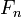# What is Gravity?

Gravitation, or gravity, is the natural phenomenon by which physical bodies attract each other with a force proportional to their masses, and inversely proportional to the square of the distance between them. It is most commonly experienced as the agent that gives weight to objects with mass and causes them to fall to the ground when dropped. The phenomenon of gravitation itself, however, is a byproduct of a more fundamental phenomenon described bygeneral relativity, which suggests that spacetime is curved according to the energy andmomentum of whatever matter and radiation are present.

In mechanics, the normal force(occasionally N) is the component, perpendicular to the surface (surface being a plane) of contact, of the contact force exerted on an object by, for example, the surface of a floor or wall, preventing the object from penetrating the surface.

The normal force is one of the components of the ground reaction force and may coincide with it, for example considering a person standing still on the ground, in which case the ground reaction force reduces to the normal force. In another common situation, if an object hits a surface with some speed, and the surface can withstand it, the normal force provides for a rapid deceleration, which will depend on the flexibility of the surface.

In kinematics, the speed of an object is the magnitude of its velocity (the rate of change of its position); it is thus a scalar quantity. The average speed of an object in an interval of time is the distance travelled by the object divided by the duration of the interval; the instantaneous speed is the limit of the average speed as the duration of the time interval approaches zero.

Like velocity, speed has the dimensions of a length divided by a time; the SI unit of speed is the metre per second, but the most usual unit of speed in everyday usage is the kilometre per hour or, in the USA and the UK, miles per hour. For air and marine travel the knot is commonly used.

The fastest possible speed at which energy or information can travel, according to special relativity, is the speed of light in a vacuum c = 299,792,458 metres per second, approximately 1079 million kilometres per hour (671,000,000 mph). Mattercannot quite reach the speed of light, as this would require an infinite amount of energy. In relativity physics, the concept of rapidity replaces the classical idea of speed.
In kinematics, velocity is the rate of change of the position of an object, equivalent to a specification of its speed and direction of motion. Speed describes only how fast an object is moving, whereas velocity gives both how fast and in what direction the object is moving. If a car is said to travel at 60 km/h, its speed has been specified. However, if the car is said to move at 60 km/h to the north, its velocity has now been specified.

To have a constant velocity, an object must have a constant speed in a constant direction. Constant direction constrains the object to motion in a straight path (the object’s path does not curve). Thus, a constant velocity means motion in a straight line at a constant speed. If there is a change in speed, direction, or both, then the object is said to have a changing velocity and is undergoing an acceleration. For example, a car moving at a constant 20 kilometres per hour in a circular path has a constant speed, but does not have a constant velocity because its direction changes. Hence, the car is considered to be undergoing an acceleration.

Velocity is a vector physical quantity; both magnitude and direction are required to define it. The scalar absolute value (magnitude) of velocity is called “speed”, a quantity that is measured in metres per second (m/s or m⋅s−1) when using the SI(metric) system. For example, “5 metres per second” is a scalar (not a vector), whereas “5 metres per second east” is a vector. The rate of change of velocity (in m/s) as a function of time (in s) is “acceleration” (in m/s2 – stated “metres per second per second”), which describes how an object’s speed and direction of travel change at each point in time. In science a “deceleration” is called a “negative acceleration”, for example: −2 m/s2.

Tags : Physicsscience